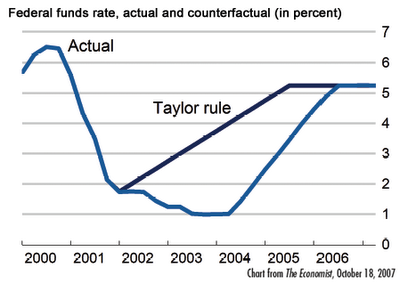Wednesday, May 13, 2009

Taylor Rule and Interest Rates

The Taylor rule is a formula for setting interest rates depending on changes in the inflation rate and economic growth.

A simplified formula is: r = p + 0.5y + 0.5 (p - 2) + 2 (after Tobin, 1998)
• r = the short term interest rate in percentage terms per annum.
• p = the rate of inflation over the previous four quarters.
• y = the difference between real GDP from potential output.
• This assumes that target inflation is 2% and equilibrium real interest rate is 2%

Example of Taylor Rule:
• If inflation were to rise by 1%, the Taylor response would be to raise the interest rate by about 1.5%
• If GDP falls by 1% relative long run trend rate, then the Taylor response is to cut the interest rate by about 0.5%
• Basically, higher growth and inflationary pressures require higher interest rates to reduce economic activity. Lower growth and a fall in inflation require lower interest rates to boost spending.
Using this formula, it is clear that in the period 2002-04, the US Federal Reserve kept interest rates too low. These low interest rate were a key factor (though by no means the only cause) of a boom in credit and a boom in house prices.

Interest Rates Too Low in US Using Taylor RuleThe interesting thing is what the Taylor rule says about current interest rates. Since GDP in US has collapsed by - 4% (when growth trend is about 2%) it means that GDP is much lower than potential. Paul Krugman estimated that using the Taylor rule, the US should give a nominal interest rate of -7%. This indicates why the Federal Reserve are having to resort to quantitative easing. They can't cut interest rates below 0%, so they need to resort to unorthodox measures to boost the economy.

Taylor Rule and Suggested Interest Rate for US